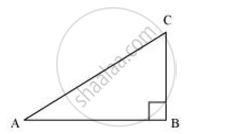Share

# In ΔABC, right angled at B. If tan A = 1/√3 , find the value of (i) sin A cos C + cos A sin C (ii) cos A cos C − sin A sin C - CBSE Class 10 - Mathematics

#### Question

In ΔABC, right angled at B. If tan A = 1/sqrt3 , find the value of

(i) sin A cos C + cos A sin C

(ii) cos A cos C − sin A sin C

#### Solutiontan A = 1/sqrt3

(BC)/(AB) = 1/ sqrt3

If BC is k, then AB will be sqrt3k , where k is a positive integer.

In ΔABC,

AC2 = AB2 + BC2

= (sqrt3k)^2 + (k)^2

= 3k2 + k2 = 4k2

∴ AC = 2k

sin A = ("Side adjacent to"angleA)/"Hypotenuse" = (BC)/(AC) = k/(2k) = 1/2

cos A = ("Side adjacent to"angleA)/"Hypotenuse" = (AB)/(AC) = (sqrt3k)/(2k) = sqrt3/2

sin C = ("Side adjacent to"angleC)/"Hypotenuse" = (AB)/(AC) = (sqrt3k)/(2k) = sqrt3/2

cos C = ("Side adjacent to"angleC)/"Hypotenuse" = (BC)/(AC) = (k)/(2k) = 1/2

(i) sin A cos C + cos A sin C

= (1/2)(1/2)+(sqrt3/2)(sqrt3/2) =  1/4 ++ 3/4

= 4/4 = 1

(ii) cos A cos C − sin A sin C

= (sqrt3/2)(1/2)-(1/2)(sqrt3/2) =  sqrt3/4 - sqrt3/4 = 0

Is there an error in this question or solution?

#### APPEARS IN

NCERT Solution for Mathematics Textbook for Class 10 (2019 to Current)
Chapter 8: Introduction to Trigonometry
Ex. 8.10 | Q: 9 | Page no. 181
Solution In ΔABC, right angled at B. If tan A = 1/√3 , find the value of (i) sin A cos C + cos A sin C (ii) cos A cos C − sin A sin C Concept: Trigonometric Ratios.
S